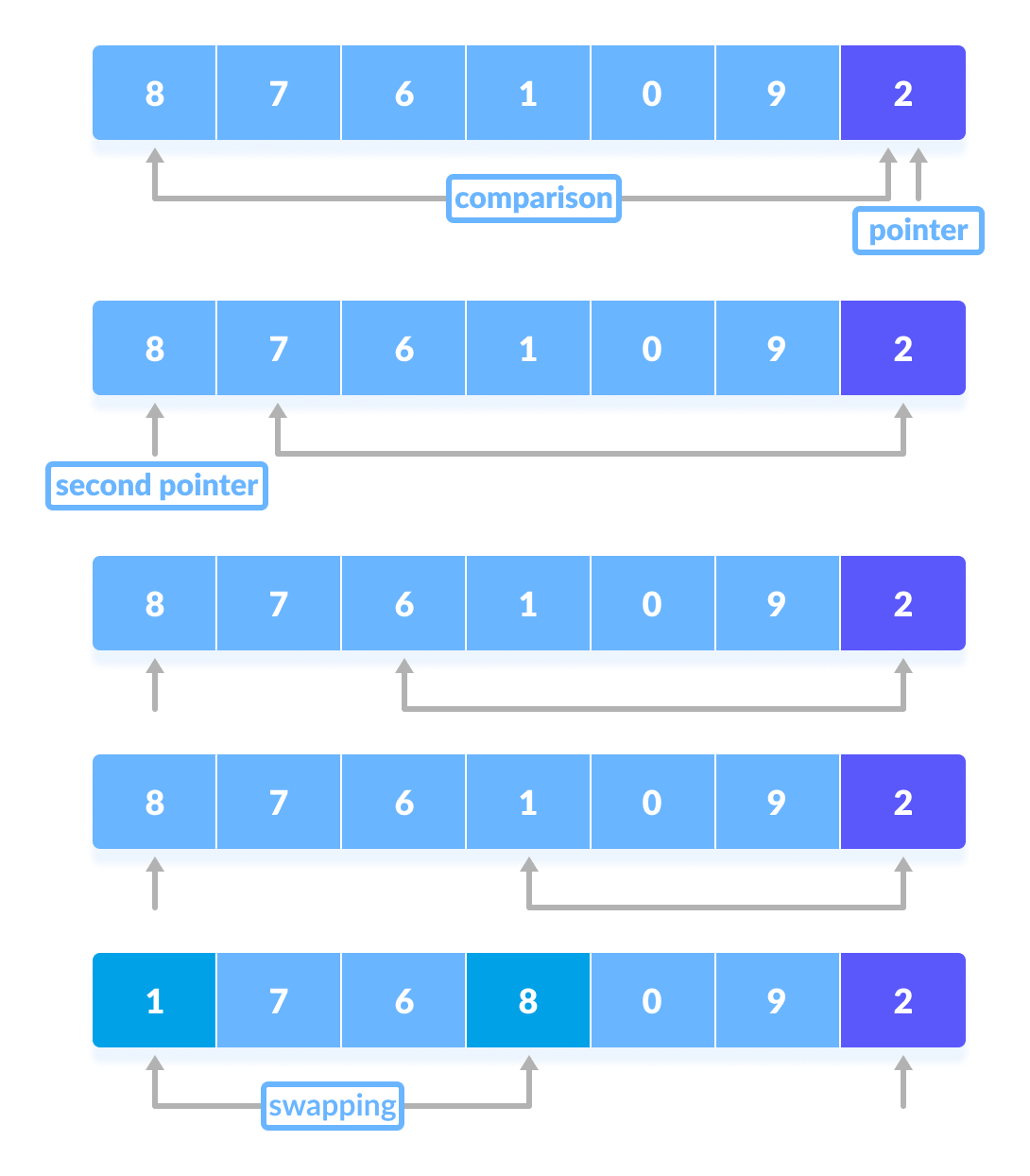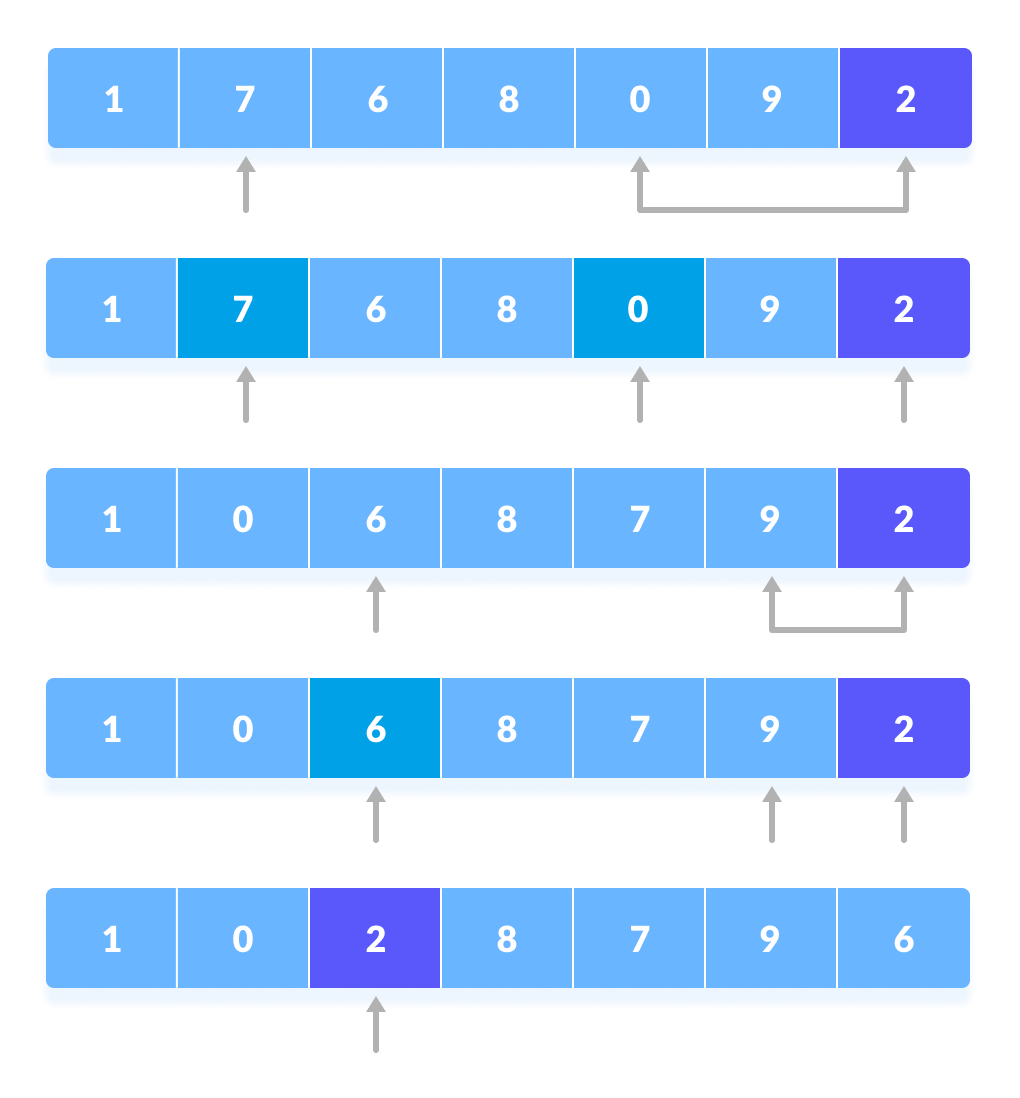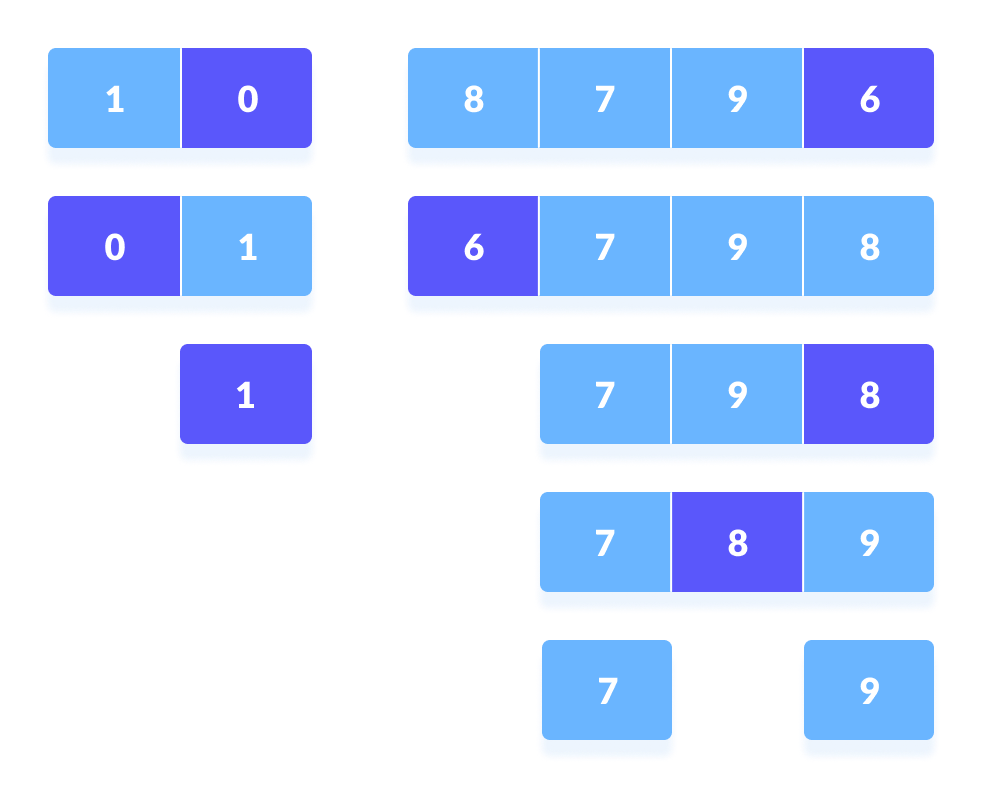# Quicksort Algorithm

#### In this tutorial, you will learn how quicksort works. Also, you will find working examples of quicksort in C, C++ Python and Java.

Quicksort is an algorithm based on divide and conquer approach in which the array is split into subarrays and these sub-arrays are recursively called to sort the elements.

## How QuickSort Works?

1. A pivot element is chosen from the array. You can choose any element from the array as the pivot element.
Here, we have taken the rightmost (ie. the last element) of the array as the pivot element.Select a pivot element
2. The elements smaller than the pivot element are put on the left and the elements greater than the pivot element are put on the right.Put all the smaller elements on the left and greater on the right of pivot element
The above arrangement is achieved by the following steps.
1. A pointer is fixed at the pivot element. The pivot element is compared with the elements beginning from the first index. If the element greater than the pivot element is reached, a second pointer is set for that element.
2. Now, the pivot element is compared with the other elements (a third pointer). If an element smaller than the pivot element is reached, the smaller element is swapped with the greater element found earlier.Comparison of pivot element with other elements
3. The process goes on until the second last element is reached.
Finally, the pivot element is swapped with the second pointer.Swap pivot element with the second pointer
4. Now the left and right subparts of this pivot element are taken for further processing in the steps below.
3. Pivot elements are again chosen for the left and the right sub-parts separately. Within these sub-parts, the pivot elements are placed at their right position. Then, step 2 is repeated.Select pivot element of in each half and put at correct place using recursion
4. The sub-parts are again divided into smaller sub-parts until each subpart is formed of a single element.
5. At this point, the array is already sorted.

Quicksort uses recursion for sorting the sub-parts.

On the basis of Divide and conquer approach, quicksort algorithm can be explained as:

• Divide
The array is divided into subparts taking pivot as the partitioning point. The elements smaller than the pivot are placed to the left of the pivot and the elements greater than the pivot are placed to the right.
• Conquer
The left and the right subparts are again partitioned using the by selecting pivot elements for them. This can be achieved by recursively passing the subparts into the algorithm.
• Combine
This step does not play a significant role in quicksort. The array is already sorted at the end of the conquer step.

You can understand the working of quicksort with the help of the illustrations below.

## Quick Sort Algorithm

```quickSort(array, leftmostIndex, rightmostIndex)
if (leftmostIndex < rightmostIndex)
pivotIndex <- partition(array,leftmostIndex, rightmostIndex)
quickSort(array, leftmostIndex, pivotIndex)
quickSort(array, pivotIndex + 1, rightmostIndex)

partition(array, leftmostIndex, rightmostIndex)
set rightmostIndex as pivotIndex
storeIndex <- leftmostIndex - 1
for i <- leftmostIndex + 1 to rightmostIndex
if element[i] < pivotElement
swap element[i] and element[storeIndex]
storeIndex++
swap pivotElement and element[storeIndex+1]
return storeIndex + 1```

## Python, Java and C/C++ Examples

``````# Quick sort in Python

# Function to partition the array on the basis of pivot element
def partition(array, low, high):

# Select the pivot element
pivot = array[high]
i = low - 1

# Put the elements smaller than pivot on the left and greater
#than pivot on the right of pivot
for j in range(low, high):
if array[j] <= pivot:
i = i + 1
(array[i], array[j]) = (array[j], array[i])

(array[i + 1], array[high]) = (array[high], array[i + 1])

return i + 1

def quickSort(array, low, high):
if low < high:

# Select pivot position and put all the elements smaller
# than pivot on left and greater than pivot on right
pi = partition(array, low, high)

# Sort the elements on the left of pivot
quickSort(array, low, pi - 1)

# Sort the elements on the right of pivot
quickSort(array, pi + 1, high)

data = [8, 7, 2, 1, 0, 9, 6]
size = len(data)
quickSort(data, 0, size - 1)
print('Sorted Array in Ascending Order:')
print(data)``````
``````// Quick sort in Java

import java.util.Arrays;

class QuickSort {

// Function to partition the array on the basis of pivot element
int partition(int array[], int low, int high) {

// Select the pivot element
int pivot = array[high];
int i = (low - 1);

// Put the elements smaller than pivot on the left and
// greater than pivot on the right of pivot
for (int j = low; j < high; j++) {
if (array[j] <= pivot) {
i++;
int temp = array[i];
array[i] = array[j];
array[j] = temp;
}
}
int temp = array[i + 1];
array[i + 1] = array[high];
array[high] = temp;
return (i + 1);
}

void quickSort(int array[], int low, int high) {
if (low < high) {

// Select pivot position and put all the elements smaller
// than pivot on left and greater than pivot on right
int pi = partition(array, low, high);

// Sort the elements on the left of pivot
quickSort(array, low, pi - 1);

// Sort the elements on the right of pivot
quickSort(array, pi + 1, high);
}
}

// Driver code
public static void main(String args[]) {
int[] data = { 8, 7, 2, 1, 0, 9, 6 };
int size = data.length;
QuickSort qs = new QuickSort();
qs.quickSort(data, 0, size - 1);
System.out.println("Sorted Array in Ascending Order: ");
System.out.println(Arrays.toString(data));
}
}``````
``````// Quick sort in C

#include <stdio.h>

// Function to swap position of elements
void swap(int *a, int *b) {
int t = *a;
*a = *b;
*b = t;
}

// Function to partition the array on the basis of pivot element
int partition(int array[], int low, int high) {

// Select the pivot element
int pivot = array[high];
int i = (low - 1);

// Put the elements smaller than pivot on the left
// and greater than pivot on the right of pivot
for (int j = low; j < high; j++) {
if (array[j] <= pivot) {
i++;
swap(&array[i], &array[j]);
}
}

swap(&array[i + 1], &array[high]);
return (i + 1);
}

void quickSort(int array[], int low, int high) {
if (low < high) {

// Select pivot position and put all the elements smaller
// than pivot on left and greater than pivot on right
int pi = partition(array, low, high);

// Sort the elements on the left of pivot
quickSort(array, low, pi - 1);

// Sort the elements on the right of pivot
quickSort(array, pi + 1, high);
}
}

// Function to print eklements of an array
void printArray(int array[], int size) {
for (int i = 0; i < size; ++i) {
printf("%d  ", array[i]);
}
printf("\n");
}

// Driver code
int main() {
int data[] = {8, 7, 2, 1, 0, 9, 6};
int n = sizeof(data) / sizeof(data);
quickSort(data, 0, n - 1);
printf("Sorted array in ascending order: \n");
printArray(data, n);
}``````
``````// Quick sort in C++

#include <iostream>
using namespace std;

// Function to swap position of elements
void swap(int *a, int *b) {
int t = *a;
*a = *b;
*b = t;
}

// Function to print eklements of an array
void printArray(int array[], int size) {
int i;
for (i = 0; i < size; i++)
cout << array[i] << " ";
cout << endl;
}

// Function to partition the array on the basis of pivot element
int partition(int array[], int low, int high) {
// Select the pivot element
int pivot = array[high];
int i = (low - 1);

// Put the elements smaller than pivot on the left
// and greater than pivot on the right of pivot
for (int j = low; j < high; j++) {
if (array[j] <= pivot) {
i++;
swap(&array[i], &array[j]);
}
}
printArray(array, 7);
cout << "........\n";
swap(&array[i + 1], &array[high]);
return (i + 1);
}

void quickSort(int array[], int low, int high) {
if (low < high) {
// Select pivot position and put all the elements smaller
// than pivot on left and greater than pivot on right
int pi = partition(array, low, high);

// Sort the elements on the left of pivot
quickSort(array, low, pi - 1);

// Sort the elements on the right of pivot
quickSort(array, pi + 1, high);
}
}

// Driver code
int main() {
int data[] = {8, 7, 6, 1, 0, 9, 2};
int n = sizeof(data) / sizeof(data);
quickSort(data, 0, n - 1);
cout << "Sorted array in ascending order: \n";
printArray(data, n);
}``````

## Quicksort Complexity

Time Complexities

• Worst Case Complexity [Big-O]: `O(n2)`
It occurs when the pivot element picked is either the greatest or the smallest element.
This condition leads to the case in which the pivot element lies in an extreme end of the sorted array. One sub-array is always empty and another sub-array contains `n - 1` elements. Thus, quicksort is called only on this sub-array.
However, the quick sort algorithm has better performance for scattered pivots.

• Best Case Complexity [Big-omega]: `O(n*log n)`
It occurs when the pivot element is always the middle element or near to the middle element.
• Average Case Complexity [Big-theta]: `O(n*log n)`
It occurs when the above conditions do not occur.

Space Complexity

The space complexity for quicksort is `O(log n)`.

## Quicksort Applications

Quicksort is implemented when

• the programming language is good for recursion
• time complexity matters
• space complexity matters